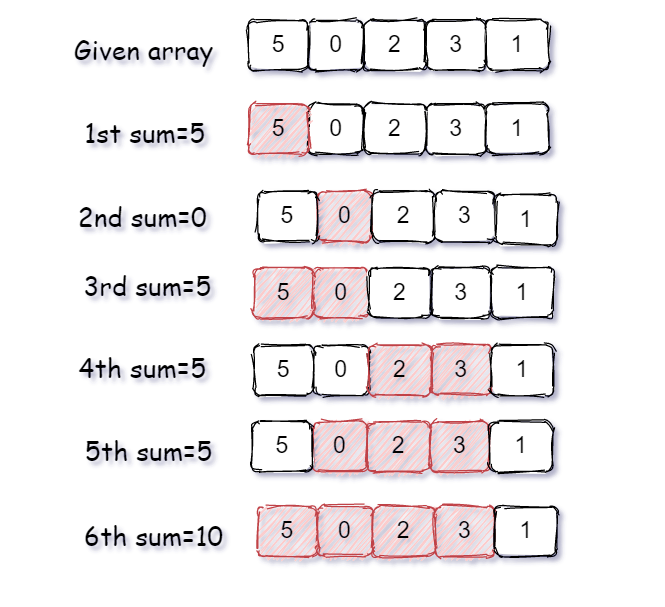# Count all sub-arrays having sum divisible by k

Posted: 8 Dec, 2020
Difficulty: Moderate

## PROBLEM STATEMENT

#### Given an array ‘ARR’ and an integer ‘K’, your task is to find all the count of all sub-arrays whose sum is divisible by the given integer ‘K’.

##### Note:
``````If there exists no subarray in the given sequence whose sum is divisible by ‘K’ then simply return ‘0’.
``````
##### Example:
``````Suppose the given array is ‘ARR’ = { 5, 0, 2, 3, 1} and ‘K = 5’.
As we can see in below image, there are a total of 6 subarrays that have the total sum divisible by ‘K’
So we return the integer 6.
``````##### Input format:
``````The first line of input contains an integer ‘T’ denoting the number of test cases.
The next ‘2*T’ lines represent the ‘T’ test cases.

The first line of each test case contains two space-separated integers the first integer ‘N’ will denote the number of elements in the array and the second integer denotes integer ‘K’.

The second line of each test case contains ‘N’ space-separated integer that is elements of the array.
``````
##### Output Format
``````For each test case, print an integer that is the count of all subarray that sum is divisible by ‘K’.
``````
##### Note:
``````You are not required to print the output explicitly, it has already been taken care of. Just implement the function.
``````
##### Constraints:
``````1 <= T <= 50
1 <= K,N <= 10^4
-10^9 <= ARR[i] <= 10^9

Time limit: 1 second
``````Approach 1

The basic idea is that try each and every possible subarray and find the sum of the current subarray and check if the current sum is divisible by ‘K’.

• To implement this approach we use two nested loops and one ‘COUNT’ variable to store all subarray with sum divisible by ‘K’ and initially ‘COUNT’ is ‘0’.
• Iterate outer loop ‘i’ from ‘0’ to ‘N-1’ for every position of ‘ARR’.
• Add check current sum is divisible by ‘K’
• If divisible then increase ‘COUNT’ by ‘1’.
• Iterate an inner loop ‘j’ from ‘i’ to ‘N-1’ and ‘j’ will represent a subarray from ‘i' to ‘j’
• Store sum of subarray from ‘i’ to ‘j’ in a ‘CUR_SUM’ variable.